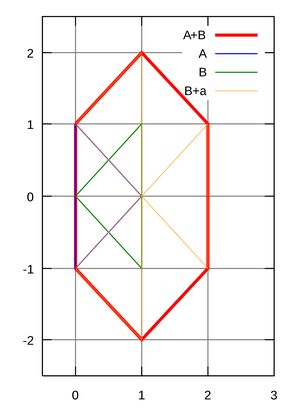# Glossary

## Minkowski sum

The Minkowski sum of two multisets $S_1$ and $S_2$ is the multiset $S_1 \oplus S_2$ formed by taking the collection of all possible sums $s_1 + s_2$, where $s_1$ is an element of $S_1$ and $s_2$ is an element of $S_2$.